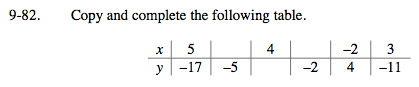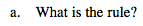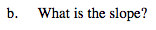### Home > MC2 > Chapter 9 > Lesson 9.3.1 > Problem9-82

9-82.Since no x-value of 0 is given, we will have to solve for the rule by finding the slope and then any possible constants.

From Chapter 7, we know that slope is the following:

$\frac{\text{change in{\it y}\;values}}{\text{change in{\it x} values}}$

Choosing any two given set of x- and y- values, we can find the slope of the equation. For example, choosing (5, −17) and (3, −11):

$\frac{\text{change in{\it y} values}}{\text{change in{\it x} values}} = \frac{-6}{2} = -3$

So far, the rule is y = −3x. Test this rule to see if it is complete by substituting in a value of x and checking if the correct y-value is given. What do you notice? Is there another constant value that is part of the rule?

y = −3x − 2
Use this rule to fill in the table.What is the slope you solved for the rule in part (a)?Explore the problem with the etool below.
Click the link at right for the full version of the eTool: MC2 9-82 HW eTool